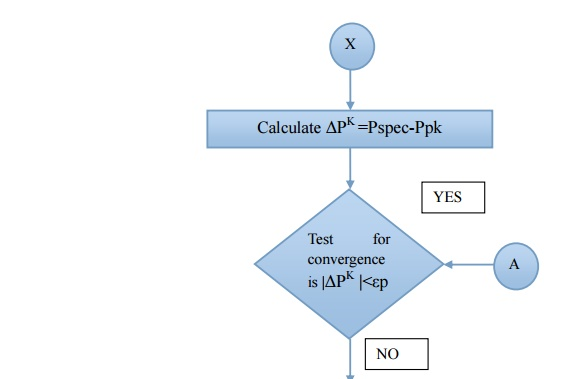Home | | Power System Analysis | Iterative Solution Using Fast Decoupled Load Flow Method - Algorithm

# Iterative Solution Using Fast Decoupled Load Flow Method - Algorithm

Step 1: Assume a suitable solution for all buses except the slack bus. Let Vp =1+j0 for P=2,3,...................n and V=a+j0

ITERATIVE SOLUTION USING FAST DECOUPLED LOAD FLOW METHOD - ALGORITHM

Step 1: Assume a suitable solution for all buses except the slack bus. Let Vp =1+j0 for P=2,3,...................n and V=a+j0

Step2: Set the convergence criterion = ε0

Step3: Set iteration count K = 0

Step 4: Set bus count P = 2

Step 5: Calculate Pp and Qp usingStep 6: Compute the real and reactive power mismatches ΔPK and ΔQK. If the mismatches Are with in desirable tolerance the iteration end

Step 7: Normalize the mismatches by dividing each entry by its respective bus voltage

magnitude ΔPK =ΔP 2K / V 2KStep 8: Solve for the voltage magnitude and the correction factors ΔVK and δK by using the constant matrices B’ and B” which are extracted from the bus admittance matrix Y Bus

[B’] δK  = ΔPK

[B”]ΔQK   = ΔQK

Step 9: Up date the voltage magnitude and angel vectors

δK+1 = δK δK

VK+1 = VK +ΔVK

Step 10: Check if all the buses are taken into account if yes go to next step otherwise go to next step. Otherwise go to step 4

Step 11: Advance iteration count K = K+1 go to step 3

Step 12: Evaluate bus and load powers and print the resultsStudy Material, Lecturing Notes, Assignment, Reference, Wiki description explanation, brief detail
Power System Analysis : Power Flow Analysis : Iterative Solution Using Fast Decoupled Load Flow Method - Algorithm |